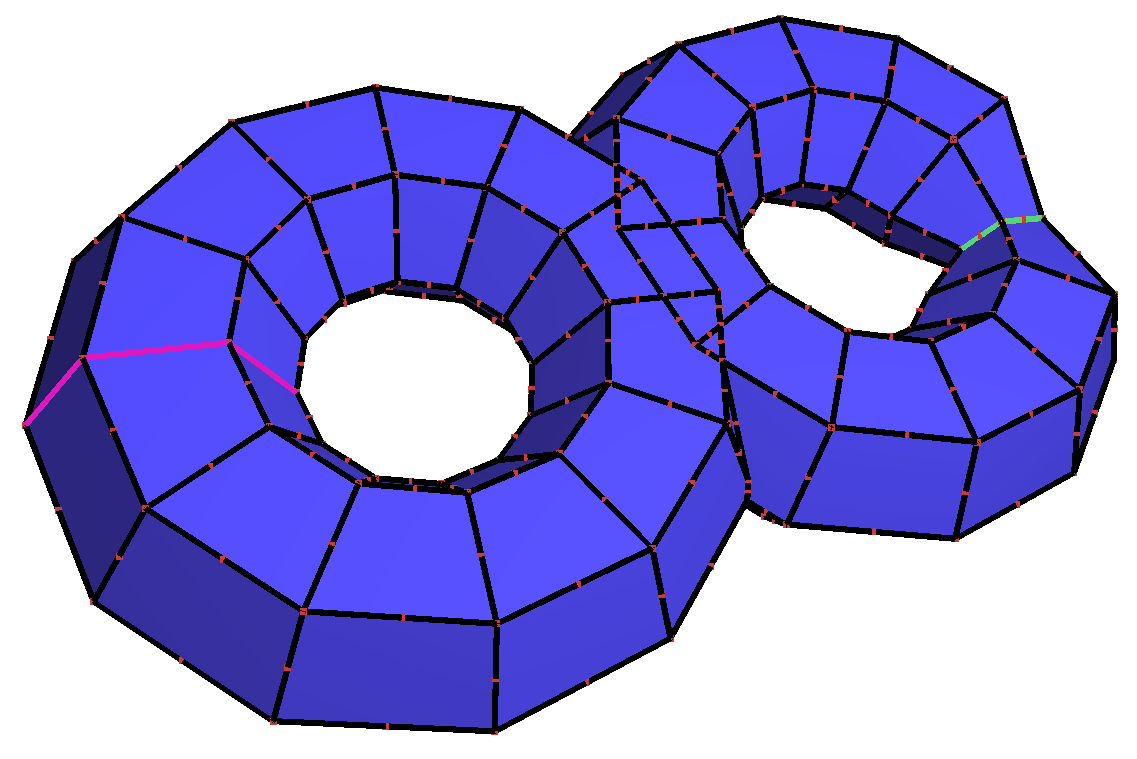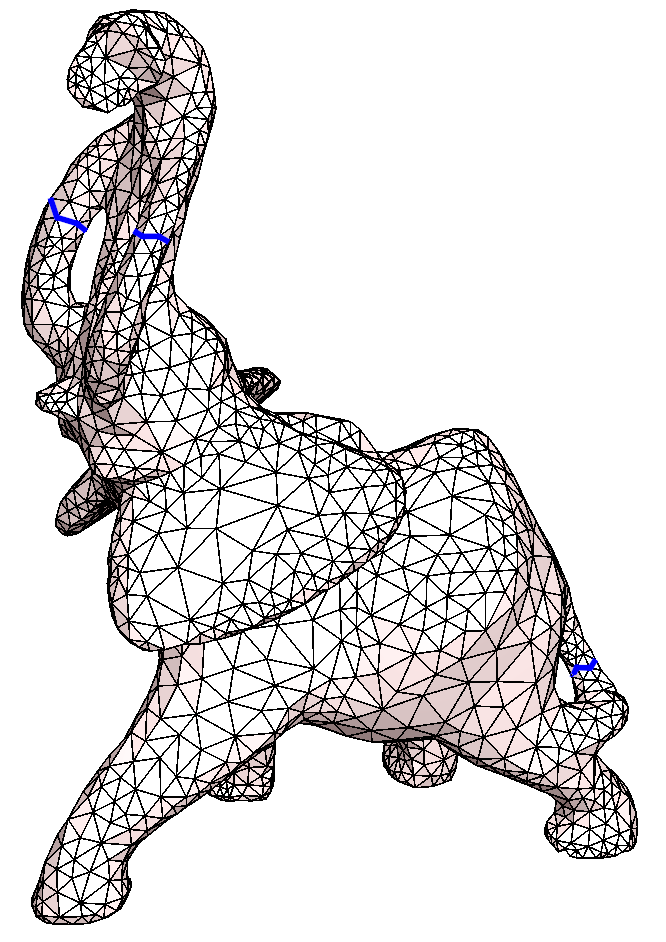# New in CGAL: Surface Mesh Topology

### Surface Mesh Topology

This new package provides a toolbox for manipulating curves on a combinatorial surface from the topological point of view. Two main functionalities are proposed. One functionality is the computation of shortest curves that cannot be continuously deformed to a point. This includes the computation of the so-called edge width and face width of the vertex-edge graph of a combinatorial surface. The other functionality is the homotopy test to decide if two given curves on a combinatorial surface can be continuously deformed one into the other.

### Computing Shortest Non-contractible Cycles

Four algorithms are implemented in the first version of this package:

• computing a shortest non-contractible cycle going through a vertex (see this function),
• computing a shortest non-contractible cycle through every vertex and returns the shortest cycle among them, possibly with weights associated with edges (see this function),
• computing the edge width of the mesh, i.e. a shortest non-contractible cycle with unit weights (see this function),
• computing a shortest non-contractible topological curve described as a circular sequence of traversed faces alternating with the vertices it passes through (see this function).Left:The pink cycle is the edge width of the mesh: the shortest (in the number of edges) non contractible cycle. The green cycle is the shortest (in length) non contractible cycle. Right: The three shortest non-contractible cycles computed successively on elephant mesh.

### Homotopy Tests

The following homotopy tests are available:

• test if a closed curve is contractible (see this function),
• test if two closed curves are freely homotopic (see this function),
• test if two paths are homotopic with fixed endpoints (see this function).On the upper left surface, the green curve is contractible. The red and blue curves share the same (green) endpoint. (Being closed, their two endpoints coincide.) These last two curves are freely homotopic as shown by the suggested continuous transformation of the blue curve.

The package Surface Mesh Topology is already integrated in CGAL's master branch on the CGAL GitHub repository, and will be officially released in the upcoming version of CGAL, CGAL 5.1, scheduled for July 2020.

Documentation of the package Surface Mesh Topology
CGAL master branch on GitHub